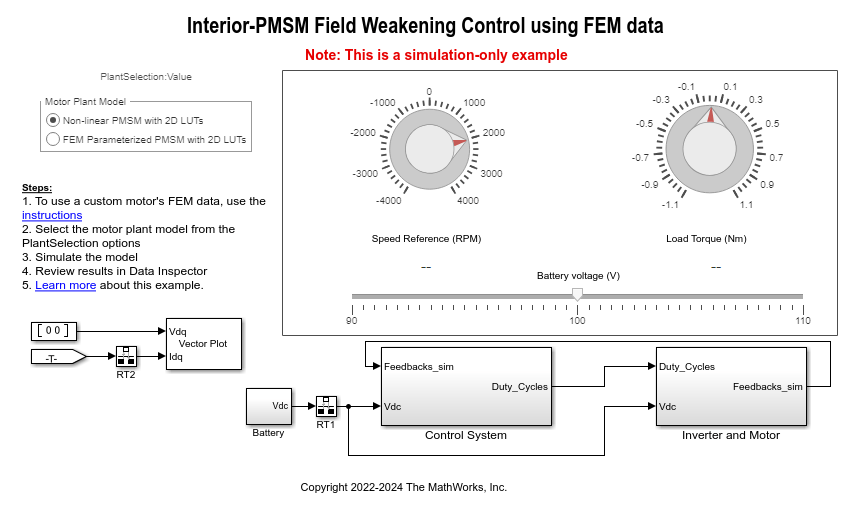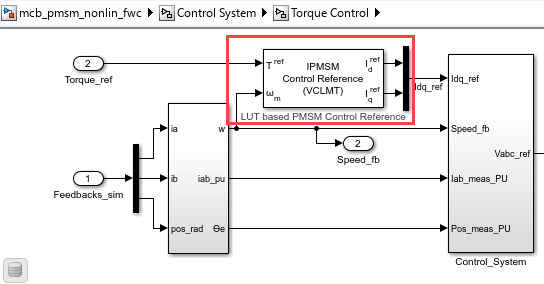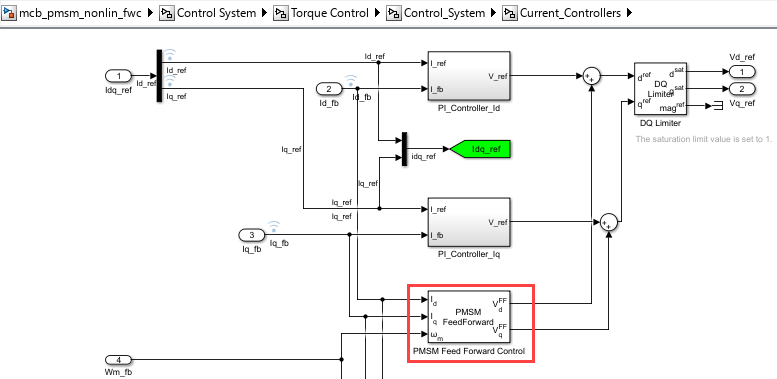# Field-Weakening Control (with MTPA) of Non-Linear PMSM Using Look-Up Table

This example uses a look-up table (LUT) for a non-linear PMSM and controller to run the motor using field-weakening control (with MTPA). Use this example to replicate and run a finite element analysis (FEA)-based non-linear high fidelity permanent magnet synchronous motor (PMSM) in simulation. This example helps motor design engineers to simulate high performance motors for real-world motor control applications. In addition, control system engineers can use this example to design control algorithms for a given set of motor parameter data to achieve high levels of accuracy in tracking and controlling speed and torque as well as meet efficiency requirements especially for high-performance motors.

The following image shows the MTPA and field-weakening operating points for an interior PMSM (IPMSM).Using an actual PMSM and hardware to design a motor as well as the control algorithm can be impractical due to higher operation costs and time, safety issues, as well as sporadic machine and inverter failures. The example effectively solves these problems by using a simulation-based model driven by an LUT that contains motor parameter data. It uses an LUT that contains `Ld` and `Lq` data with respect to currents `id` and `iq`.

Traditionally, generation of optimal reference current data would require an actual motor and hardware in a dyno setup. The example reduces this effort by using the LUT based PMSM Control Reference block that characterizes the PMSM and obtains the machine's nonlinear inductance and permanent magnet flux linkage data, which can then be used to design and implement flux-weakening torque control `id`(torque, speed), `iq`(torque, speed) LUTs by using iterative analytical methods.

By default, the example uses pre-generated and calibrated inductance and permanent magnet flux linkage LUT data sets provided by JMAG. However, as an alternative, you can configure this block and generate your own reference current `id`(torque, speed), `iq`(torque, speed) LUT and automatically use it in the same example.

You can use the example to simulate the LUT-based motor model and run tests to design the control algorithm. This enables you to run challenging tests easily and perform robust machine and control algorithm validation, which in turn reduces the time required for hardware testing and saves the overall development time.

The example model accepts pre-calibrated reference current LUT data files for non-linear PMSMs. Because solving the underlying motor equations for non-linear high-performance motors is computation-intensive, the example relies on LUTs generated by solving these (non-approximated) equations. This enables you to run control algorithms on the controller hardware with high accuracy (because LUT data mimics the actual non-linear motor characteristics).

Using this approach, you can reduce the complexities that occur when the algorithm relies on the controller hardware to solve approximated equations for non-linear PMSM.

The example helps you build a field-weakening (with MTPA) control algorithm that can compensate for the reduced torque caused by non-linearity developed due to a magnetically saturated PMSM stator core.

For more details about these operating regions, see Field-Weakening Control (with MTPA) of PMSM and PMSM Drive Characteristics and Constraint Curves.

### Model

This example includes the following Simulink® model:

mcb_pmsm_nonlin_fwc

You can use this model only for simulation. Click the preceding link or use the following open_system command to open the model:

```open_system('mcb_pmsm_nonlin_fwc.slx'); ```### Required MathWorks® Products

• Motor Control Blockset™

• Simscape™ Electrical™

By default, the example uses the following two LUT data files, which are loaded and read by the model initialization script `mcb_pmsm_nonlin_fwc_data.m` associated with the example model:

• `1k_S_C_IV.mat` — LUT from the product Simscape Electrical

• `JMAG_RTML-004_LdLq.mat` — Data generated from the inductance LUTs sourced from JMAG (for RTML-004 PMSM)

Note: Both the preceding `.mat` files include data for the same PMSM model from JMAG's model library.

For more details, see the System Parameters section of the model initialization script.

The following blocks in the example model uses the loaded LUT data:

• `mcb_pmsm_nonlin_fwc/Control System/Torque Control/LUT based PMSM Control Reference` — Uses the reference current LUTs derived from the inductance LUTs.• `mcb_pmsm_nonlin_fwc/Control System/Torque Control/Control_System/Current_Controllers/PMSM Feed Forward Control` — Uses the inductance LUTs.• `mcb_pmsm_nonlin_fwc/Inverter and Motor/Motor Model`

- Non-linear PMSM with 2D LUTs — This motor model uses a PMSM (Simscape Electrical) block from Simscape Electrical that extracts and uses 2D data (`id`, `iq`) from the loaded `.mat` file.

- FEM Parameterized PMSM 2D LUTs — This motor model uses the FEM-Parameterized PMSM (Simscape Electrical) block from Simscape Electrical that extracts and uses 2D data (`id`, `iq`) from the loaded `.mat` file.

- FEM Parameterized PMSM 3D LUTs — This motor model uses the FEM-Parameterized PMSM (Simscape Electrical) block from Simscape Electrical that extracts and uses 3D data (`imag`, `iangle`, `rotortheta`) from the loaded `.mat` file.### Simulate Model

1. Open the Simulink model `mcb_pmsm_nonlin_fwc.slx` associated with this example.

2. Use the Motor Plant Model radio button in the example model to select one of the following motor models that you want to simulate using the LUT data:

a. Non-linear PMSM with 2D LUTs

b. FEM Parameterized PMSM with 2D LUTs

c. FEM Parameterized PMSM with 3D LUTs

3. Click Run on the Simulation tab to simulate the model.

4. Set the reference speed and reference torque values using the Speed Reference (RPM) and Torque Reference (Nm) knobs.

5. Click Data Inspector on the Simulation tab to view and analyze the simulation results.

### Generate LUT Data File for Custom Motor Design

The following procedure explains the steps needed to obtain the inductance LUT data set (either manually generated or downloaded from JMAG) and use it with the same or a similar example model.

1. Obtain the `.rtt` file and RT-Viewer tool:

a. Visit JMAG-RT Model Library to download the LUT data set (`.rtt` file) for your motor, which includes the `Ld`, `Lq` maps.

b. Visit JMAG-RT Viewer to obtain the tool RT-Viewer.

2. Use the RT Viewer tool to open the `.rtt` file and export the `Ld`, `Lq` table values into an identical grid of the `id`, `iq` values. For example, save the `Ld`, `Lq` table values as `JMAG_Ld.csv`, and `JMAG_Lq.csv`.

- In the RT Viewer tool menu, select Table Value > Inductance > Id-Iq-Ld.

- In the Map Window menu, select File > CSV Export to open the CSV Export window.

- In the CSV Export window, provide `id`, `iq` grid details and the file name to proceed with the data export.

Note: If you have a manually generated LUT data set, skip steps 1 and 2.

3. In the model initialization script associated with the Simulink model, create template structures for PMSM and inverter as shown below:

`pmsm=mcb_SetPMSMMotorParameters('Teknic2310P');`

`inverter= mcb_SetInverterParameters("BoostXL-DRV8305");`

`pmsm.B=pmsm.B*100; % increasing the viscous damping coefficient since this is a bigger motor than the Teknic2310p.`

4. In the script, find the motor parameters and update the following fields:

`pmsm.p (pole pairs)`

`pmsm.Rs (stator/Coil Resistance)`

`pmsm.Ld (d_inductance)`

`pmsm.Lq (q_inductance)`

`pmsm.J (inertia)`

`pmsm.FluxPM (magflux)`

`pmsm.I_rated (rated_current)`

`inverter.V_dc (rated_voltage)`

5. Calculate the rated torque value using the following function:

`pmsm.T_rated=mcbPMSMRatedTorque(pmsm);`

6. Create a seed structure with the file paths to read the .csv files that you created in step 2.

`seed.Ldfilepath='JMAG_Ld.csv';`

`seed.Lqfilepath='JMAG_Lq.csv';`

Note: If you are using a manually generated inductance LUT data set, use the paths to the associated `.csv` files instead.

7. Create LUTs using the following API:

`PMSMLUT=mcbGenerateTables(pmsm,inverter,'jmagindfiles2inddq',seed);`

The preceding command converts the `.csv` files generated by the JMAG RT-Viewer into a regular matrix, filling the empty places using extrapolation. Because we also need the permanent magnet flux linkage (FluxPM) in the same LUT format, create an array using the following command:

`PMSMLUT.FluxPMTable=pmsm.FluxPM*ones(size(PMSMLUT.LdTable));`

8. Configure the following blocks in the example model to use the non-linear LUTs:

- LUT based PMSM Control Reference — In the block dialog box, set the parameter Motor parameter input method to `Non-linear model with id and iq LUTs`.

- PMSM Feed Forward Control — In the block dialog box, set the parameter Motor parameter input method to `Non-linear model with Ld, Lq and FluxPM LUTs`.

- PMSM Torque Estimator — In the block dialog box, set the parameter Motor parameter input method to `Non-linear model with Ld, Lq and FluxPM LUTs`.

9. In the example model, create a plant using the FEM-Parameterized PMSM block.

10. In the FEM-Parameterized PMSM block dialog box, set the Modelling option parameter to 2-D partial derivative data | No thermal port.

11. In the Electrical section of the dialog-box, set the Parameterization parameter to `Assume sinusoidal back emf - tabulate with d- and q- axis currents`.

12. To generate the `id`, `iq` LUTs from inductance LUT data, use the following command:

`PMSMLUT=mcbGenerateTables(pmsm,inverter,'idiqLUTs',seed);`

For more details about structure of the variable seed, use the MATLAB command `help mcbGenerateTables`.

13. In the model initialization script, populate the PMSM LUT fields at appropriate places depending on the parameter input method:

`PMSMLUT.idVec`

`PMSMLUT.iqVec`

`PMSMLUT.LdTable`

`PMSMLUT.LqTable`

`PMSMLUT.FluxPMTable`

or

`PMSMLUT.trefVec`

`PMSMLUT.wrpmVec`

`PMSMLUT.idTable`

`PMSMLUT.iqTable`

14. Similarly, populate the rest of the motor parameters at their respective places.

15. For the PMSM plant model:

- Maintain the default value for the stator zero sequence inductance.

- Update the variables `pmsm.B` and `pmsm.J` in the mechanical parameters section.

- Set initial targets for rotor speed and rotor position to `0`.